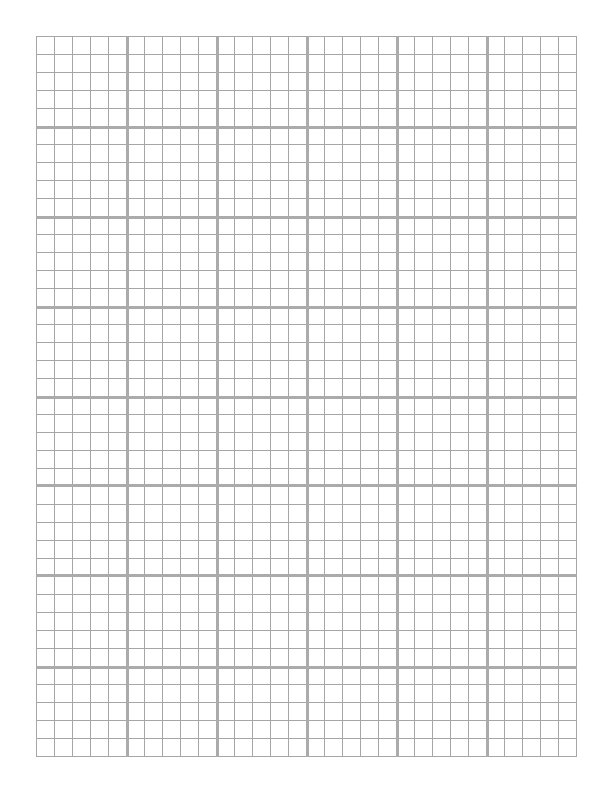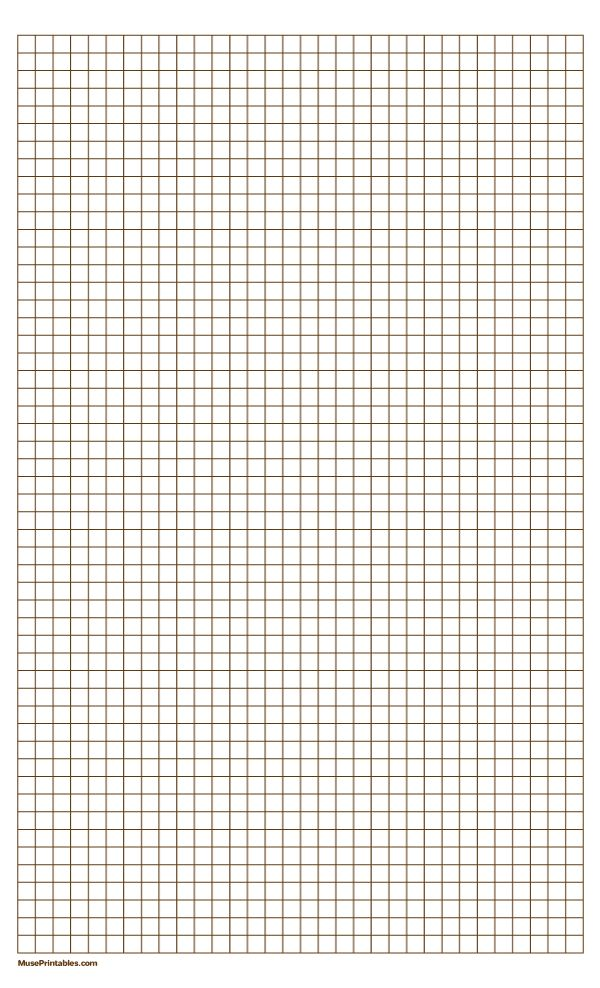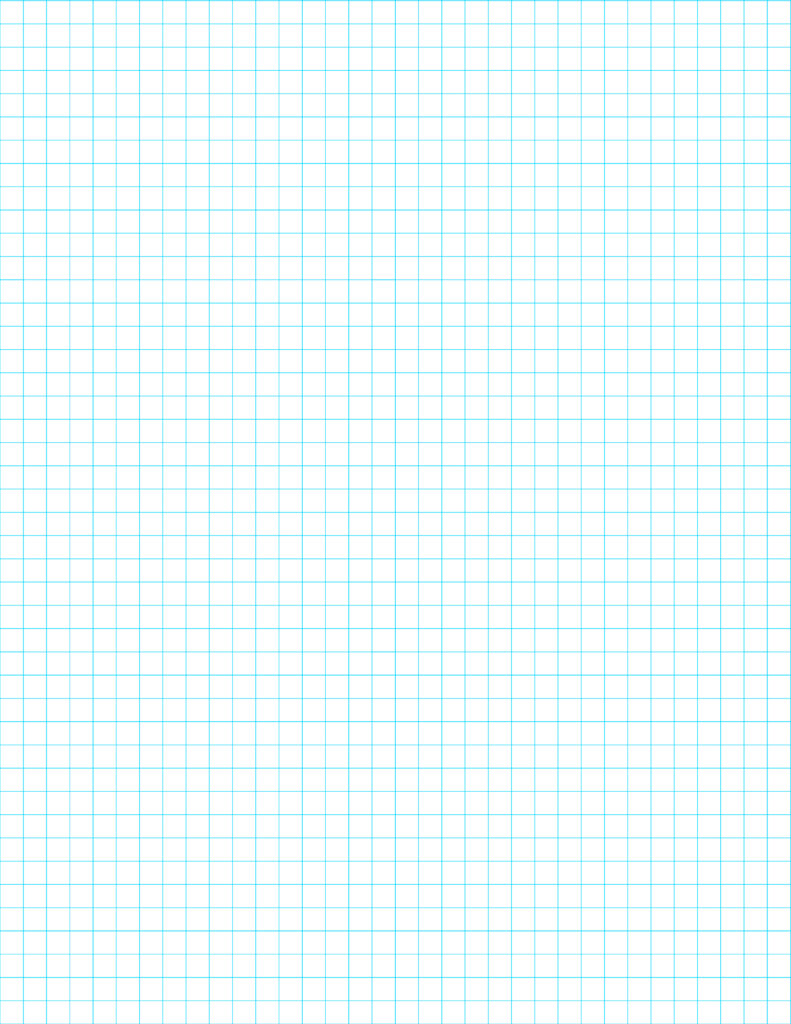# Printable Graph Paper 1 4 Inch PDF – Free Template

Graph Papers come with different grid sizes and on this page, we have shared graph paper templates with 1/4-inch grid sizes. The 1/4 inch graph paper is made of continuous small squares in a single sheet of paper. The grid size is identical throughout the graph paper and this type of paper is used by students while learning mathematics, used for technical drawings, etc. Just like any other graph paper, this type of graph paper also has its own usage and purpose. People who are looking for Printable Graph Paper 1 4 Inch templates can download the graphing paper from this page.

This type of graph paper is also referred to as quadrille paper because four boxes make up an inch. The size of the grids is what makes graphing paper different from others. This graph paper type finds its usage in mathematics, technical drawing, making cross-stitch patterns, knitting work, etc. Today in this article, we have shared 1/4 Inch Graph Paper so that you can solve your mathematical equation more easily.

Contents

## Printable Graph Paper 1 4 Inch

Graphs are commonly used to represent data or numbers in a more easy-to-understand way. You must have seen graphs while watching sports or while seeing the weather telecast on a news channel. The whole purpose of making a graph or chart is to represent the information in an engaging way so that everyone can understand it easily. Graph papers are used to make graphs or charts, now different types of graphing papers are available these days. Through this post, we are covering one of the most used graphing papers i.e. 1/4 inch printable graph paper.

### 1/4 inch printable graph paper

Graphs or charts are made in almost all the fields where it is required to present the information or number. A normal person understands graphs much better than a number sheet. Apart from the pictorial representation of data, graph paper is commonly used for drawing, making patterns, knitting, solving a mathematical problems, etc. Information or data is presented accurately with the help of graph paper and this is the reason why people use it.PDF

Mathematics, engineering, and arts are the field where you can see the major use of graph paper. An artist draws his object or patterns using graph paper. Similarly, a civil engineer uses graph paper to make the design of any building or bridge or mall, etc. Similarly, a mathematician use graph paper for solving a mathematical problem.

### graph paper 1/4 inch printable

The grid size of 1/4 graphing paper is a quarter of 1-inch graph paper, the grid size is smaller which means this paper could be used for work where data should be presented accurately. Students and working professionals who are in need of this type of graphing paper can download one from here.PDF

### free printable 1/4 inch graph paperPDF